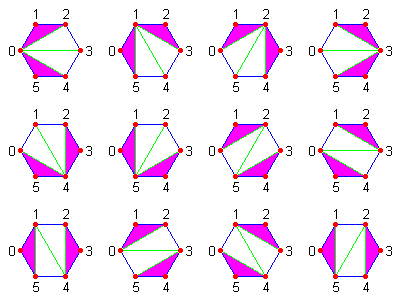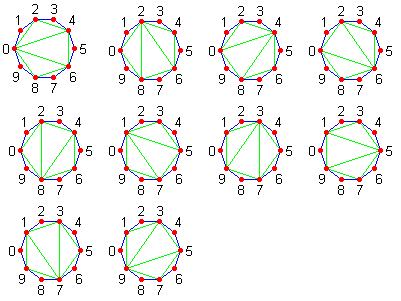### Problem Statement

Let's consider a regular polygon on the plane with n vertices, numbered from 0 to n-1, inclusive. The polygon's n-3 diagonals are called a triangulation if no two diagonals intersect at a point strictly inside the polygon. Two triangulations A and B are considered to be distinct if there are two vertices i and j such that A contains the diagonal between vertices i and j, and B doesn't contain this diagonal.

It's not hard to see that every triangulation breaks the polygon into n-2 triangles. The triangulation is called k-isosceles, if there are exactly k isosceles triangles among them. Given ints n and k, compute the number of distinct k-isosceles triangulations of a regular polygon with n vertices. Return the result modulo 123456789.

### Definition

 Class: IsoscelesTriangulations Method: getCount Parameters: int, int Returns: int Method signature: int getCount(int n, int k) (be sure your method is public)

### Notes

-A triangle is called isosceles if it has at least 2 sides with equal length.
-A regular polygon is a polygon which is equiangular (all angles are congruent) and equilateral (all sides have the same length).
-A diagonal is a line joining two nonadjacent vertices of a polygon.

### Constraints

-n will be between 3 and 50, inclusive.
-k will be between 0 and n-2, inclusive.

### Examples

0)

 `4` `2`
`Returns: 2`
 We can have a diagonal between vertices 0 and 2 or between vertices 1 and 3. In both cases, there are 2 isosceles triangles.
1)

 `3` `0`
`Returns: 0`
 The only triangulation of an equilateral triangle contains no diagonals and 1 isosceles triangle (the polygon itself).
2)

 `5` `3`
`Returns: 5`
 A regular pentagon has 5 triangulations. Each of them is obtained by connecting one selected vertex with the two others that are not its neighbors, so each triangulation is 3-isosceles.
3)

 `6` `2`
`Returns: 12`
 All 2-isosceles triangles of a regular hexagon are shown in the picture below. All isosceles triangles in each triangulation are colored purple.4)

 `10` `8`
`Returns: 10`
 All 8-isosceles triangulations of a regular 10-gon are shown in the picture below. All triangles in each triangulation are isosceles.#### Problem url:

http://www.topcoder.com/stat?c=problem_statement&pm=8179

#### Problem stats url:

http://www.topcoder.com/tc?module=ProblemDetail&rd=10785&pm=8179

ivan_metelsky

#### Testers:

PabloGilberto , Yarin , Olexiy

#### Problem categories:

Dynamic Programming